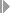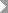Show TOC

###(12) Inventory Determination, Reserves for Follow-Up Costs, Without Milestone Billing#### Use

You can use the method Inventory Determination, Reserves for Follow-Up Costs, Without Milestone Billing for:

• Sales orders

• Projects

You can use the method Inventory Determination, Reserves for Follow-Up Costs, Without Milestone Billing to:

• Create inventories in the amount of the actual costs if the actual revenue is zero

• Create cost of sales in the amount of the planned costs if the actual revenue is not zero and the actual costs are less than the planned costs

In this case the system creates reserves for unrealized costs in the amount of the difference between the planned costs and the actual costs.

• Create cost of sales in the amount of the actual costs if the actual revenue is not zero and the actual costs are greater than the planned costs

• Once the cost of sales has been calculated, it is no longer possible to create capitalized costs.

Note the following:

• You can create reserves for unrealized costs.

• You cannot use this method with milestone billing.

• There is no proportionality between cost of sales and revenue:

As soon as revenue is received in a period, the revenue is compared either with the cumulative actual costs incurred up to that period or with the planned costs. In other words, the cost of sales is not proportional to the actual revenues as with the revenue-based method, nor is it proportional to the quantity sold as with the quantity-based method.

#### Prerequisites

You have planned costs.

Choose a results analysis method in simplified Customizing for Product Cost by Sales Order underPeriod-End ClosingResults AnalysisValuation Method.

#### Features

If R(a) = 0 then C(PA) = 0

If R(a) <> 0 and C(p) > C(a) then C(PA) = C(p)

C(r) = C(p) - C(a)

If R(a) <> 0 and C(a) > C(p) then C(PA) = C(a)

R(PA) = R(a)

If R(a) = 0 then C(z) = C(a)

If R(a) <> 0 then C(z) = 0

#### Example

Suppose you have planned costs of USD 120,000.

Period 01

In period 01 you have actual costs of USD 20,000 but no revenues. In results analysis, the system calculates the following data:

• Cost of sales affecting net income of USD 0

• Revenue affecting net income of USD 0

• Capitalized costs of USD 20,000

You then settle the following:

• Capitalized costs to FI and EC-PCA

• No cost of sales and no revenues to CO-PA

The following values are reported in CO-PA:

Profitability Analysis

 Actual revenues 0 Calculated cost of sales 0 Profit 0

The income statement shows the following values:

Income Statement

 Expense Revenue Actual costs 20,000 Inventory increase Capitalized costs 20,000 20,000 20,000

Period 02

In period 02 actual costs increase to USD 80,000. You deliver to your customer and send an invoice for USD 100,000. The order is partially delivered and partially billed. In results analysis, the system calculates the following data:

• Cost of sales affecting net income in the amount of the planned costs of USD 120,000

• Revenue affecting net income of USD 100,000

• Reserves for unrealized costs of USD 40,000

• Capitalized costs of USD 0

You then settle the following:

• Cost of sales to CO-PA

• Revenues to CO-PA

• The cancellation of the capitalized costs to FI and EC-PCA

• The reserves for unrealized costs to FI and EC-PCA

The following values are reported in CO-PA:

Profitability Analysis

 Revenues (actual revenues) 100,000 Cost of sales (planned costs) 120,000 Profit -20,000

The income statement shows the following values:

Income Statement

 Expense Revenue Actual costs 80,000 Actual revenues 100,000 Reserves for unrealized costs 40,000 Loss 20,000 120,000 120,000

Period 03

In period 03 actual costs increase to USD 130,000. You do not invoice. In results analysis, the system calculates the following data:

• Cost of sales affecting net income in the amount of the actual costs of USD 130,000

• Revenues affecting net income in the amount of the actual revenues of USD 100,000

You then settle the following:

• Cost of sales to CO-PA

• Revenues to CO-PA

The following values are reported in CO-PA:

Profitability Analysis

 Revenues (actual revenues) 100,000 Cost of sales (actual costs) 130,000 Profit - 30,000

The income statement shows the following values:

Income Statement

 Expense Revenue Actual costs 130,000 Actual revenues 100,000 Loss 30,000 130,000 130,000

Period 04

In period 04 actual costs increase to USD 140,000. You deliver to your customer and send an invoice for USD 100,000. Total revenue is USD 200,000. The order is now fully delivered and fully invoiced. In results analysis, the system calculates the following data:

• Cost of sales affecting net income in the amount of the actual costs of USD 140,000

• Revenue affecting net income of USD 200,000

You then settle the following:

• Cost of sales to CO-PA

• Revenues to CO-PA

The following values are reported in CO-PA:

Profitability Analysis

 Revenues (actual revenues) 200,000 Cost of sales (actual costs) 140,000 Profit 60,000

The income statement shows the following values:

Income Statement

 Expense Revenue Actual costs 140,000 Actual revenues 200,000 Profit 60,000 200,000 200,000

The profit on your sales order is USD 60,000.